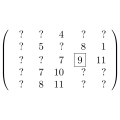CGAL 5.1.3 - Monotone and Sorted Matrix Search
Monotone and Sorted Matrix Search ReferenceMichael Hoffmann
This package provides a matrix search framework, which is the underlying technique for the computation of all furthest neighbors for the vertices of a convex polygon, maximal k-gons inscribed into a planar point set, and computing rectangular p-centers.
Introduced in: CGAL 1.1
BibTeX: cgal:h-msms-21a

This chapter describes concepts, classes, and functions for monotone and sorted matrix search.

## Assertions

The optimization code uses infix OPTIMISATION in the assertions, e.g. defining the compiler flag CGAL_OPTIMISATION_NO_PRECONDITIONS switches precondition checking off, cf. Section Checks in the chapter on STL extensions.

## Classified Reference Pages

• CGAL::monotone_matrix_search
• CGAL::Dynamic_matrix<M>
• MonotoneMatrixSearchTraits
• BasicMatrix
• CGAL::sorted_matrix_search
• CGAL::Sorted_matrix_search_traits_adaptor<F,M>
• SortedMatrixSearchTraits

Concepts

## Classes

class  CGAL::Dynamic_matrix< M >
The class Dynamic_matrix is an adaptor for an arbitrary matrix class M to provide the dynamic operations needed for monotone matrix search. More...

The class Sorted_matrix_search_traits_adaptor can be used as an adaptor to create sorted matrix search traits classes for arbitrary feasibility test and matrix classes F resp. M. More...

## Functions

template<class Matrix , class RandomAccessIC , class Compare_strictly >
void CGAL::monotone_matrix_search (const Matrix &m, RandomAccessIC t, const Compare_strictly &compare_strictly=less< Matrix::Value >())
computes the maximum (as specified by compare_strictly) entry for each row of m and writes the corresponding column to t, i.e., t[i] is set to the index of the column containing the maximum element in row i. The maximum $$m_r$$ of a row $$r$$ is the leftmost element for which compare_strictly $$(m_r,\,x)$$ is false for all elements $$x$$ in $$r$$. More...

template<class RandomAccessIterator , class Traits >
Traits::Value CGAL::sorted_matrix_search (RandomAccessIterator f, RandomAccessIterator l, const Traits &t)
returns the element x in one of the sorted matrices from the range [f, l), for which t.is_feasible(x) is true and t.compare(x, y) is true for all other y values from any matrix for which t.is_feasible(y) is true. More...

## ◆ monotone_matrix_search()

template<class Matrix , class RandomAccessIC , class Compare_strictly >
 void CGAL::monotone_matrix_search ( const Matrix & m, RandomAccessIC t, const Compare_strictly & compare_strictly = less< Matrix::Value >() )

#include <CGAL/monotone_matrix_search.h>

computes the maximum (as specified by compare_strictly) entry for each row of m and writes the corresponding column to t, i.e., t[i] is set to the index of the column containing the maximum element in row i. The maximum $$m_r$$ of a row $$r$$ is the leftmost element for which compare_strictly $$(m_r,\,x)$$ is false for all elements $$x$$ in $$r$$.

The function monotone_matrix_search() computes the maxima for all rows of a totally monotone matrix.

More precisely, monotony for matrices is defined as follows.

Let $$K$$ be a totally ordered set, $$M \in K^{(n,\, m)}$$ a matrix over $$K$$ and for $$0 \le i < n$$:

$rmax_M(i) :\in \left\{ \min_{0 \le j < m} j \: \left|\: M[i,\, j] = \max_{0 \le k < m} M[i,\, k] \right.\right\}$

the (leftmost) column containing the maximum entry in row $$i$$. $$M$$ is called monotone, iff

$\forall\, 0 \le i_1 < i_2 < n\: :\: rmax_M(i_1) \le rmax_M(i_2)\; .$

$$M$$ is totally monotone, iff all of its submatrices are monotone (or equivalently: iff all $$2 \times 2$$ submatrices are monotone).

Precondition
t points to a structure of size at least m.number_of_rows()
Template Parameters
 Matrix is a model of MonotoneMatrixSearchTraits. RandomAccessIC is a model of RandomAccessIterator with int as value type. If compare_strictly is defined, it is an adaptable binary function: Matrix::Value $$\times$$ Matrix::Value $$\rightarrow$$ bool describing a strict (non-reflexive) total ordering on Matrix::Value.
MonotoneMatrixSearchTraits
all_furthest_neighbors_2()
maximum_area_inscribed_k_gon_2()
maximum_perimeter_inscribed_k_gon_2()
extremal_polygon_2()

Implementation

The implementation uses an algorithm by Aggarwal et al.. The runtime is linear in the number of rows and columns of the matrix.

## ◆ sorted_matrix_search()

template<class RandomAccessIterator , class Traits >
 Traits::Value CGAL::sorted_matrix_search ( RandomAccessIterator f, RandomAccessIterator l, const Traits & t )

#include <CGAL/sorted_matrix_search.h>

returns the element x in one of the sorted matrices from the range [f, l), for which t.is_feasible(x) is true and t.compare(x, y) is true for all other y values from any matrix for which t.is_feasible(y) is true.

The function sorted_matrix_search() selects the smallest entry in a set of sorted matrices that fulfills a certain feasibility criterion.

More exactly, a matrix $$M = (m_{i j}) \in S^{r \times l}$$ (over a totally ordered set $$S$$) is sorted, iff

\begin{eqnarray*} \forall \, 1 \le i \le r,\; 1 \le j < l\; :\; m_{i j} \le m_{i (j+1)} \;\; {\it and}\\ \forall \, 1 \le i < r,\; 1 \le j \le l\; :\; m_{i j} \le m_{(i+1) j} \;\;. \end{eqnarray*}

Now let $$\mathcal{M}$$ be a set of $$n$$ sorted matrices over $$S$$ and $$f$$ be a monotone predicate on $$S$$, i.e.\

$f\: :\: S \longrightarrow\, \textit{bool} \quad{\rm with}\quad f(r) \;\Longrightarrow\; \forall\, t \in S\,,\: t > r \; :\; f(t)\;.$

If we assume there is any feasible element in one of the matrices in $$\mathcal{M}$$, there certainly is a smallest such element. This is the one we are searching for.

The feasibility test as well as some other parameters can (and have to) be customized through a traits class.

Precondition
1. All matrices in $$\left[f,\, l\right)$$ are sorted according to Traits::compare_non_strictly.
2. There is at least one entry $$x$$ in a matrix $$M \in \left[f,\, l\right)$$ for which Traits::is_feasible(x) is true.
Template Parameters
 Traits is a model for SortedMatrixSearchTraits. RandomAccessIterator has Traits::Matrix as value type.

Implementation

The implementation uses an algorithm by Frederickson and Johnson,  and runs in $$\mathcal{O}(n \cdot k + f \cdot \log (n \cdot k))$$, where $$n$$ is the number of input matrices, $$k$$ denotes the maximal dimension of any input matrix and $$f$$ the time needed for one feasibility test.

SortedMatrixSearchTraits